# Samacheer Kalvi 12th Accountancy Solutions Chapter 9 Ratio Analysis

Students can Download Accountancy Chapter 9 Ratio Analysis Questions and Answers, Notes Pdf, Samacheer Kalvi 12th Accountancy Book Solutions Guide Pdf helps you to revise the complete Tamilnadu State Board New Syllabus and score more marks in your examinations.

## Tamilnadu Samacheer Kalvi 12th Accountancy Solutions Chapter 9 Ratio Analysis

### Samacheer Kalvi 12th Accountancy Ratio Analysis Text Book Back Questions and Answers

Question 1.
The mathematical expression that provides a measure of the relationship between two figures is called ……………..
(a) Conclusion
(b) Ratio
(c) Model
(d) Decision
(b) Ratio

Question 2.
Current ratio indicates ……………..
(a) Ability to meet short term obligations
(b) Efficiency of management
(c) Profitability
(d) Long term solvency
(a) Ability to meet short term obligationsQuestion 3.
Current assets excluding inventory and prepaid expenses is called ……………..
(a) Reserves
(b) Tangible assets
(c) Funds
(d) Quick assets
(d) Quick assets

Question 4.
Debt equity ratio is a measure of ……………..
(a) Short term solvency
(b) Long term solvency
(c) Profitability
(d) Efficiency
(b) Long term solvency

Question 5.
Match List I with List II and select the correct answer using the codes given below: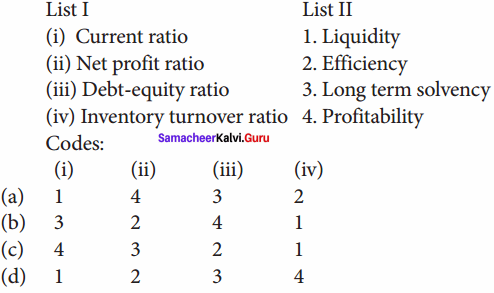(a) 1 4 3 2

Question 6.
To test the liquidity of a concern, which of the following ratios are useful?
(i) Quick ratio
(ii) Net profit ratio
(iii) Debt – equity ratio
(iv) Current ratio
Select the correct answer using the codes given below:
(a) (i) and (ii)
(b) (i) and (iv)
(c) (ii) and (iii)
(d) (ii) and (iv)
(b) (i) and (iv)

Question 7.
Proportion of share holder’s funds to total assets is called ……………..
(a) Proprietary ratio
(b) Capital gearing ratio
(c) Debt equity ratio
(d) Current ratio
(a) Proprietary ratio

Question 8.
Which one of the following is not correctly matched?
(a) Liquid ratio – Proportion
(b) Gross profit ratio – Percentage
(c) Fixed assets turnover ratio – Percentage
(d) Debt – equity ratio – Proportion
(c) Fixed assets turnover ratio – PercentageQuestion 9.
Current liabilities ₹ 40,000; Current assets ₹ 1,00,000 ; Inventory ₹ 20,000 . Quick ratio is ……………..
(a) 1:1
(b) 2.5:1
(c) 2:1
(d) 1:2
(c) 2:1

Question 10.
Cost of revenue from operations ₹ 3,00,000; Inventory in the beginning of the year ₹ 60,000; Inventory at the close of the year ₹ 40,000. Inventory turnover ratio is
(a) 2 times
(b) 3 times
(c) 6 times
(d) 8 times
(c) 6 times

Question 1.
What is meant by accounting ratios?
Ratio is a mathematical expression of relationship between two related or interdependent items. It is the numerical or quantitative relationship between two items. It is calculated by dividing one item by the other related item. When ratios are calculated on the basis of accounting information, these are called ‘accounting ratios’.

Question 2.
What is quick ratio?
Quick ratio gives the proportion of quick assets to current liabilities. It indicates whether the business concern is in a position to pay its current liabilities as and when they become due, out of its quick assets. Quick assets are current assets excluding inventories and prepaid expenses. It is otherwise called “liquid ratio” or “acid test ratio”.
Quick ratio = $$\frac{\text { Quick assets }}{\text { Current liabilities }}$$
Quick assets = Current assets – Inventories – Prepaid expenses.
Higher the quick ratio, better is the short – term financial position of an enterprise.Question 3.
What is meant by debt equity ratio?
It is calculated to assess the long term solvency position of a business concern. Debt equity ratio expresses the relationship between long term debt and shareholder’s funds.
Debt equity ratio = $$\frac{\text { Long term debt }}{\text { Shareholders funds }}$$
Capital employed = Shareholder’s funds + Non current liabilities
Greater the return on investment better is the profitability of a business and vice versa.

Question 4.
What does return on investment ratio indicate?
It shows the proportion of net profit before interest and tax to capital employed (shareholder’s fund and long term debts). It is an overall measure of profitability of a business concern.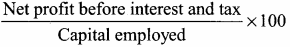Capital employed = Shareholder’s funds + Non current liabilities
Greater the return on investment better is the profitability of a business and vice versa.

Question 5.
State any two limitations of ratio analysis.
Ratios are only means: Ratios are not end in themselves but they are only means to achieve a particular purpose. Analysis of related items must be done by the management or experts with the help of ratios. Change in price level: Ratio analysis may not reflect price level changes and current values as they are calculated based on historical data given in financial statement.

Question 1.
Explain the objectives of ratio analysis.
The objectives of ratio analysis:

1. To simplify accounting figures
2. To facilitate analysis of financial statements
3. To analysis the operational efficiency of a business
4. To help in budgeting and forecasting
5. To facilitate intra firm and inter firm comparison of performance

Question 2.
What is inventory conversion period? How is it calculated?
It is the time taken to sell the inventory. A shorter inventory conversion period indicates more efficiency in the management of inventory. It is computed as follows: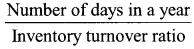(in days)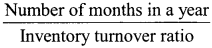(in months)

Question 3.
How is operating profit ascertained?
It gives the proportion of operating profit to revenue from operations. Operating profit ratio is an indicator of operational efficiency of an organisation. It may be computed as follows: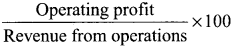Alternatively, it is calculated as under:
Operating profit ratio = 100 – Operating cost ratio
Operating profit = Revenue from operations – Operating cost

Question 4.
State any three advantages of ratio analysis.
1. Measuring operational efficiency: Ratio analysis helps to know operational efficiency of a business by finding the relationship between operating cost and revenues and also by comparison of present ratios with those of the past ratios.

2. Intra firm comparison: Comparison of efficiency of different divisions of an organisation is possible by comparing the relevant ratios.

3. Inter firm comparison: Ratio analysis helps the firm to compare its performance with other firms.Question 5.
Bring out the limitations of ratio analysis.
Following are the limitations of ratio analysis:
1. Ratios are only means: Ratios are not end in themselves but they are only means to achieve a particular purpose. Analysis of related items must be done by the management or experts with the help of ratios.

2. Accuracy of financial information: The accuracy of a ratio depends on the accuracy of information taken from financial statements. If the statements are inaccurate, ratios computed based on that will also be inaccurate.

3. Consistency in preparation of financial statements: Inter firm comparisons with the help of ratio analysis will be meaningful only if the firms follow uniform accounting procedures consistently.

4. Non – availability of standards or norms: Ratios will be meaningful only if they are compared with accepted standards or norms. Only few financial ratios have universally recognised standards. For other ratios, comparison with standards is not possible.

5. Change in price level: Ratio analysis may not reflect price level changes and current values as they are calculated based on historical data given in financial statements.

IV. Exercises

Question 1.
Calculate the current ratio from the following information.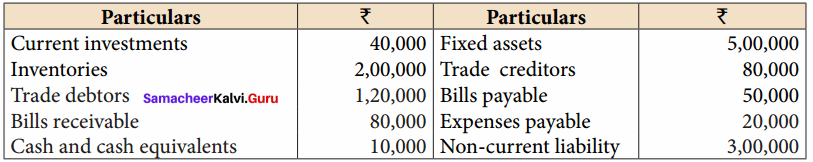Solution:
Current ratio =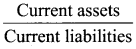Current assets = current investment + Inventories + Trade debtors + Bills receivable + Cash and cash equivalents
C. A = 40,000 + 2,00,000 + 1,20,000 + 80,000 + 10,000 = 4,50,000
Current liablities = Trade crditors + Bills payable + Expenses payable
C.L = 80,000 + 50,000 + 20,000
= 1,50,000
Current ratio = $$\frac { 4,50,000 }{ 1,50,000 }$$
Current ratio = 3:1

Question 2.
Calculate quick ratio: Total current liabilities ₹ 2,40,000; Total current assets ₹ 4,50,000; Inventories ₹ 70,000; Prepaid expenses ₹ 20,000
Solution:
Quick ratio =Quick ratio = Current assets – Inventory – Prepaid expenses = 4,50,000 – 70,000 – 20,000
= 3,60,000
Quick ratio = $$\frac { 3,60,000 }{ 2,40,000 }$$ = 1.5 : 1

Question 3.
Following is the balance sheet of Lakshmi Ltd. as on 31st March, 2019: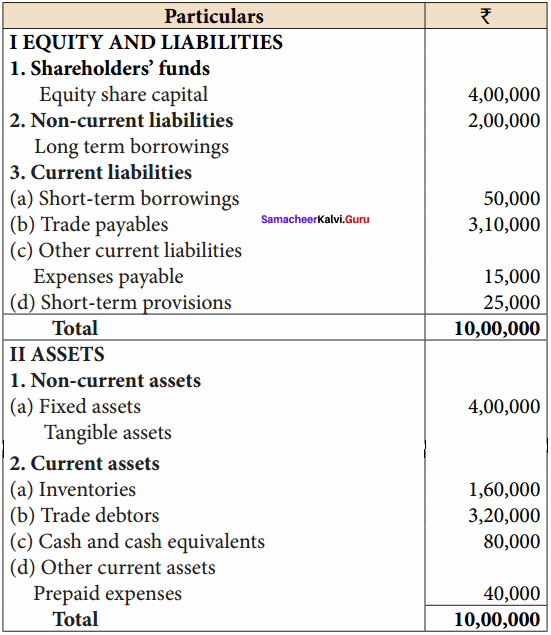Calculate:
(i) Current ratio (ii) Quick ratio
Solution:
(i) Current ratio == $$\frac { 6,00,000 }{ 4,00,000 }$$
Current ratio = 1.5:1
Current assets = Inventories + Trade debtors + Cash and cash equivalents + other current assets prepaid expenses.
= 40,000 + 1,60,000 + 3,20,000 + 80,000
= 6,00,000
Current Liabilities = short-term loans + trade payables + Expenses payable + short term provision.
= 50,000 + 3,10,000 + 15,000 + 25,000
= 4,00,000
Quick ratio = $$\frac{\text { Quick assets }}{\text { Current liabilities }}$$
Quick assets = Current assets – Inventory – Prepaid expenses
= 6,00,000 – 1,60,000 – 40,000 = 4,00,000
Quick ratio = $$\frac { 4,00,000 }{ 4,00,000 }$$ = 1 : 1

Question 4.
From the following information calculate debt equity ratio.
Balance sheet (Extract) as on 31st March, 2019: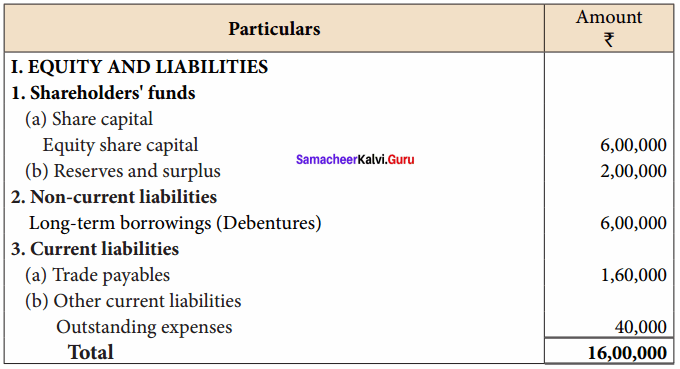Solution:
Debt equity ratio = $$\frac{\text { Long term debt }}{\text { Shareholders funds }}$$
Debentures = 6,00,000
Shareholders’ funds = Equity share capital + Reserves and surplus
= 6,00,000 + 2,00,000
= 8,00,000
Debt Equity ratio = $$\frac { 6,00,000 }{ 8,00,000 }$$ = 0.75 : 1

Question 5.
From the following Balance Sheet of Pioneer Ltd. calculate proprietary ratio:
Balance sheet of Pioneer Ltd. as on 31.3.2019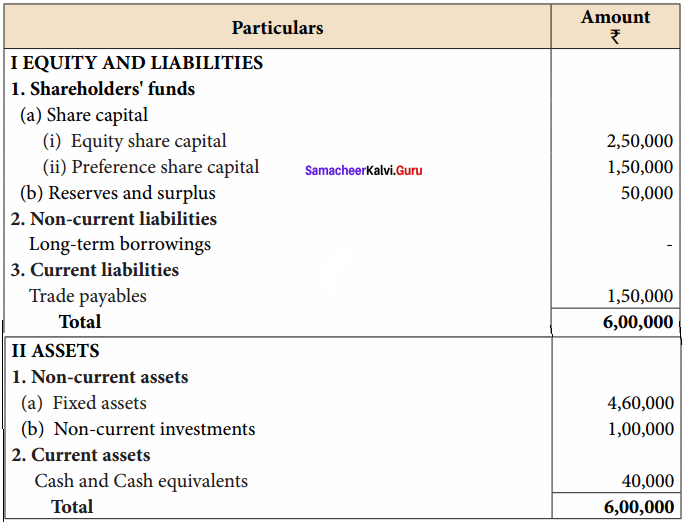Solution: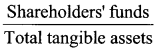Shareholders’ funds = Equity share capital + Preference share capital + Reserves and surplus
= 2,50,000 + 1,50,000 + 50,000
= 4,50,000
Total tanginle assets = Fixed assets capital + preference share capital + Reserves and surplus
= 2,50,000 + 1,00,000 + 40,000
=6,00,000
Proprietary Ratio = $$\frac { 4,50,000 }{ 6,00,000 }$$ = 0.75 : 1

Question 6.
From the following information calculate capital gearing ratio:
Balance Sheet (Extract) as on 31.03.2018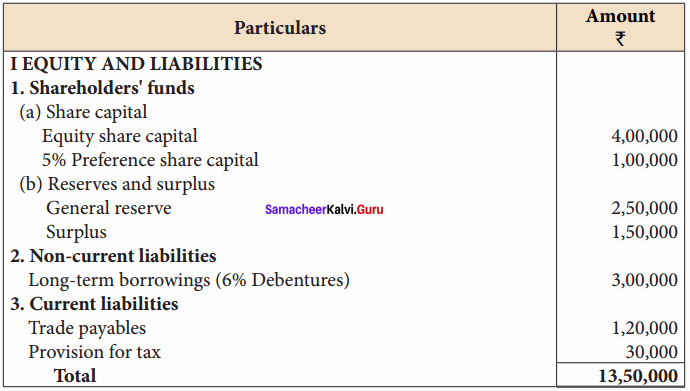Solution: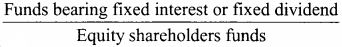Capital gearing ratio =0.5 : 1
= $$\frac { 4,00,000 }{ 8,00,000 }$$
Funds bearing fixed interest and dividend Preference share capital + Debentures
= 1,00,000 + 3,00,000 = 4,00,000
Equity shareholder’s funds = Equity share capital + General reserve + Surplus
= 4,00,000 + 2,50,000 + 1,5,000 = 8,00,000

Question 7.
From the following Balance Sheet of Arunan Ltd. as on 31.03.2019 calculate

1. Debt – equity ratio
2. Proprietary ratio
3. Capital gearing ratio

Balance Sheet of Arunan Ltd. as on 31.03.2019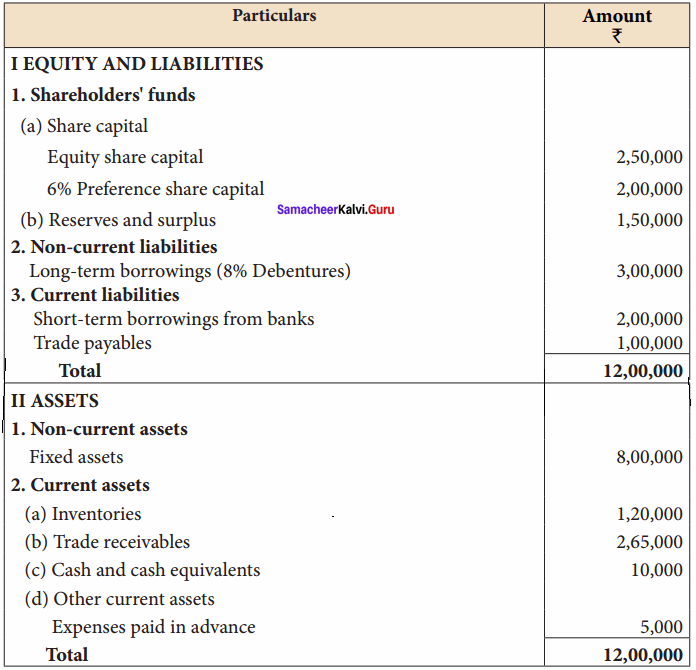Solution:
1. Debt Equity Ratio = $$\frac{\text { Long term debt }}{\text { Shareholders funds }}$$
Long term debt = Debentures = 3,00,000
Shareholders’ funds = Equity share capital + Preference share capital + Reserves and surplus
= 2,50,000 + 2,00,000 + 1 ,50,000
= 6,00,000
Debt Equity Ratio = $$\frac { 3,00,000 }{ 6,00,000 }$$ = 0.5 : 1
Debt – equity ratio : 0.5 : 1

2. Proprietary ratio =Total Tangible assets = 12,00,000
Proprietary ratio = $$\frac { 6,00,000 }{ 12,00,000 }$$ = 0.5 : 1

3. Capital geanng ratio =Funds bearing fixed interest or dividend = Preference share capital + Debentures + Long term borrowings.
= 2,00,000 + 4,00,000 = 5,00,000
Equity shareholders’ funds = Equity share capital + Reserves and surplus
= 2,50,000 + 1,50,000 = 4,00,000
Capital gearing ratio = $$\frac { 5,00,000 }{ 4,00,000 }$$ = 1.25 : 1

Question 8.
From the given information calculate the inventory turnover ratio and inventory conversion period (in months) of Sania Ltd.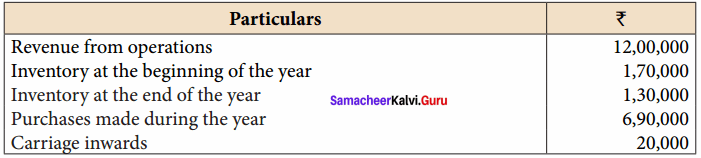Solution:
Inventory turnover ratio =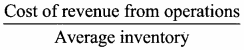Cost of revenue from operations = Opening inventory + Net Purchases + Direct expenses (carriage inwards) – Closing inventory
= 1,70,000 + 6,90,000 + 20,000 – 1,30,000
= 7,50,000
Average inventory =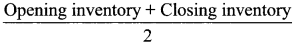= 1,50,000
Inventory Turnover ratio = $$\frac { 7,50,000 }{ 1,50,000 }$$ = 5 times
Inventory conversion period =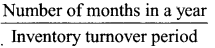= $$\frac { 12 }{ 5 }$$ 2.4 months

Question 9.
The credit revenue from operations of Harini Ltd. amounted to ₹ 9,60,000. Its debtors and bills receivable at the end of the accounting period amounted to ₹ 1,00,000 and ₹ 60,000, respectively. Calculate trade receivable turnover ratio and also collection period in months.
Solution:
Trade receivables turnover ratio =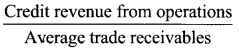Average trade Receivables = Debtors + Bills receivable
Average Trade Receivables = $$\frac{1,10,000+1,40,000}{2}$$ = 2,50,000
Trade receivable turnover ratio = $$\frac{ 10,00,000}{2,50,000}$$ = 4 times
Debt collection period =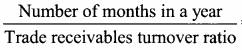= 3 months.

Question 10.
From the following figures obtained from Kalpana Ltd, calculate the trade payables turnover ratio and credit payment period (in days).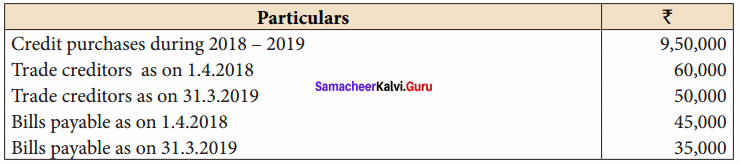Solution:
Trade payable turnover ratio =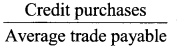Average trade payable =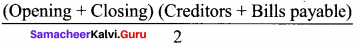= $$\frac{60,000+50,000+45,000+35,000}{2}$$
= 95,000
Trade payable turnover ratio = $$\frac{9,50,000}{95,000}$$ = 10 times
Credit payment period =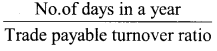= $$\frac{365}{10}$$ = 36.5 days

Question 11.
From the following information of Ashika Ltd., calculate fixed assets turnover ratio:

1. Revenue from operations during the year were ₹ 60,00,000.
2. Fixed assets at the end of the year was ₹ 6,00,000.

Solution:
Fixed assets turnover ratio =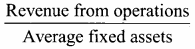= $$\frac{ 55,00,000}{ 5,00,000}$$ = 11 times

Question 12.
Calculate:

1. Inventory turnover ratio
3. Trade payable turnover ratio and
4. Fixed assets turnover ratio from the following information obtained from Delphi Ltd.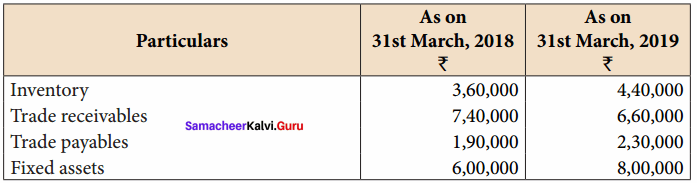1. Revenue from operations for the year ₹ 10,50,000
2. Purchases for the year ₹ 4,50,000
3. Cost of revenue from operations ₹ 6,00,000. Assume that sales and purchases are for credit.

Solution:
1. Inventory turnover ratio =Average inventory =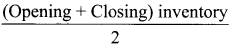= $$\frac{3,60,000+4,40,000}{2}$$ = 4,00,000
Inventory turnover ratio = $$\frac{ 16,00,000}{4,00,000}$$ = 4 times

2. Trade receivables turnover ratio =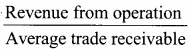Average trade receivable = $$\frac{ 7,40,000 + 6,60,000 }{2}$$ = 7,00,000

Trade receivables turnover ratio = $$\frac{ 35,00,000 }{ 7,00,000}$$ = 5 times

3. Trade payable turnover ratio =Average trade receivable = $$\frac{ 1,90,000 + 2,30,000 }{2}$$ = 21,00,000
Trade payable turnover ratio = $$\frac{ 21,00,000}{2,10,000}$$ = 10 times

4. Fixed assets turnover ratio =Average fixed assets = $$\frac{ 6,00,000 + 8,00,000 }{2}$$ = 7,00,000
Fixed assets turnover ratio = $$\frac{ 35,00,000}{7,00,000}$$ = 5 times

Question 13.
Calculate gross profit ratio from the following:
Revenue from operations ₹ 1,00,000, Cost of revenue from operations ₹ 80,000 and purchases ₹ 62,500.
Solution:
Gross profit ratio =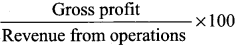Gross profit = Revenue from operations – Cost of revenue from operations
= 2,50,000 – 2,10,000
= 40,000
Greater profit ratio = $$\frac{40,000}{2,50,000}$$ x 100 = 16%

Question 14.
Following is the statement of profit and loss of Maria Ltd. for the year ended 31st March, 2018. Calculate the operating cost ratio.
Statement of Profit and Loss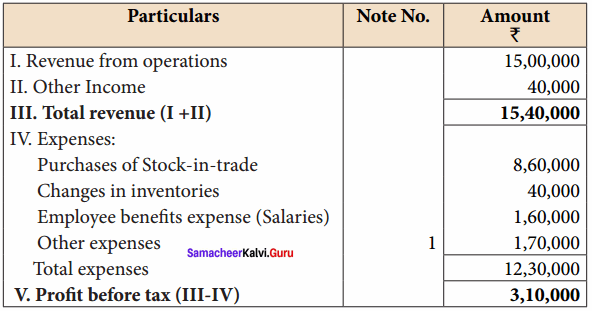Notes to Accounts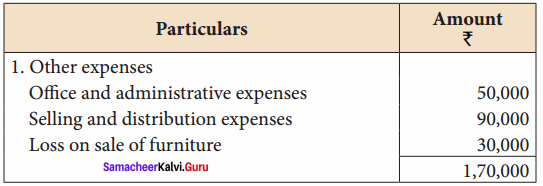Solution:
Operating cost ratio =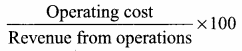Cost of revenue from operations = Purchases of stock-in-trade + Change in inventories of stock
= 8,60,000 + 40,000 = 9,00,000
Operating expenses = office and administrative expenses + Selling and distribution expenses + Employee benefits expenses salaries
= 50,000 + 90,000 + 1,60,000 = 3,00,000
Operating cost = Cost of revenue from operations + Operating expenses
= 9,00,000 + 3,00,000
= 12,00,000
Operating cost ratio = $$\frac{12,00,000}{15,00,000}$$ x 100 = 80%

Question 15.
Calculate operating profit ratio under the following cases.
Case 1: Revenue from operations ₹ 10,00,000, Operating profit ₹ 1,50,000.
Case 2: Revenue from operations ₹ 15,00,000, Operating cost ₹ 12,00,000.
Case 3: Revenue from operations ₹ 20,00,000, Gross profit 30% on revenue from operations, Operating expenses ₹ 4,00,000
Solution:
Operating ratio =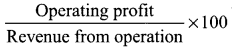Case I :
Operating ratio = $$\frac{2,00,000}{8,00,000}$$ x 100 = 25%

Case II.
Operating profit = Revenue from operation – Operating Cost
= 20,00,000 – 14,00,000 = 6,00,000
Operating ratio = $$\frac{6,00,000}{20,00,000}$$ x 100 = 30%

Case III.
Operating profit = Gross profit – Operating expenses
Gross profit = 10,00,000 x $$\frac{ 25 }{ 100 }$$ = 2,50,000
Operating profit = 2,50,000 – 1,00,000 = 1,50,000
Operating ratio = $$\frac{1,50,000}{10,00,000}$$ x 100 = 1.5%

Question 16.
From the following details of a business concern calculate net profit ratio.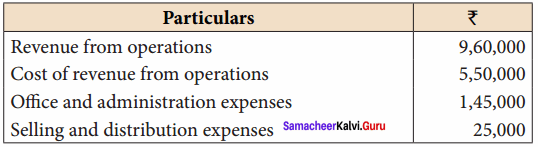Solution:
Net Profit Ratio =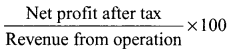Net profit = Revenue from operations – Cost of revenue from operation – office and administrative expenses – selling and distribution expenses
=9,60,000 – 5,50,000 – 1,45,000 – 1,25,000 = 2,40,000
Net profit ratio = $$\frac{2,40,000}{9,60,000}$$ x 100 = 25%

Question 17.
From the following statement of profit and loss of Mukesh Ltd. calculate

1. Gross profit ratio
2. Net profit ratio.

Statement of Profit and Loss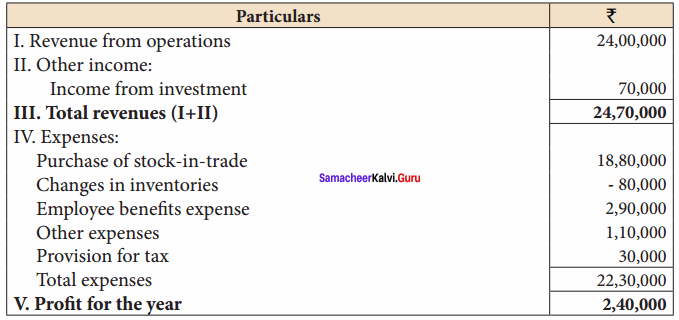Solution:
1. Gross Profit =Gross profit = Revenue from operations – Purchase of stock in trade – Changes in inventories
= 24,00,000 – 18,80,000 – ( – 80,000)
= 6,00,000
Greater profit ratio = $$\frac{6,00,000}{24,00,000}$$ x 100 = 10%

2. Net Profit Ratio =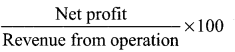Net profit ratio = $$\frac{2,40,000}{24,00,000}$$ x 100 = 10%

Question 18.
From the following trading activities of Naveen Ltd. calculate

1. Gross profit ratio
2. Net profit ratio
3. Operating cost ratio
4. Operating profit ratio

Statement of Profit and loss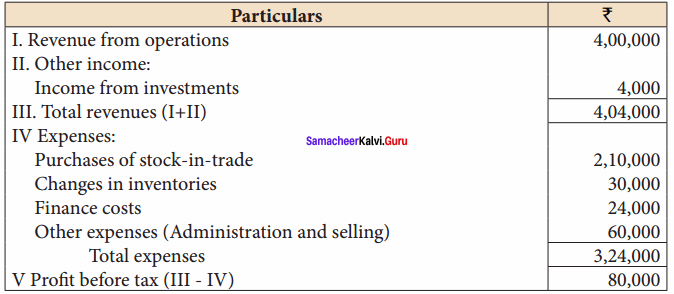Solution:
1. Gross profit ratio =Gross profit = Revenue from operations – Purchase of stock in trade – Changes in inventories
= 44,00,000 – 21,00,000 – 30,000
= 16,00,000
Greater profit ratio = $$\frac{1,60,000}{4,00,000}$$ x 100 = 40%

2. Net Profit Ratio =Net profit ratio = $$\frac{80,000}{4,00,000}$$ x 100 = 20%

3. Operating cost ratio =Cost of revenue from operations = Purchases of stock-in-trade + Change in inventories of stock
= 2,10,000 + 30,000 = 2,40,000
Operating expenses = Administrative expenses + Selling and expenses = 60,000
Operating cost = 2,40,000 + 60,000 = 3,00,000
Operating cost ratio = $$\frac{3,00,000}{4,00,000}$$ x 100 = 75%

4. Operating profit ratio =Operating profit = Gross profit – Operating expenses
= 1,60,000 – 60,000 = 1,00,000
Operating profit ratio = $$\frac{10,00,00}{4,00,000}$$ x 100 = 25%

Question 19.
Following is the extract of the balance sheet of Babu Ltd., as on 31st March, 2018: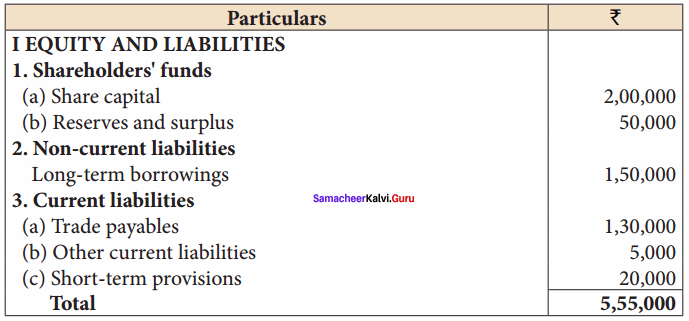Net profit before interest and tax for the year was ₹ 25,000. Calculate the return on capital employed for the year.
Solution: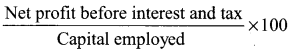Capital employed = Share capital + Reserves and surplus + Long term borrowings
= 2,00,000 + 50,000 + 1,50,000 = 4,00,000
Return on Capital employed = $$\frac{60,000}{4,00,000}$$ x 100 = 15%

Question 1.
What are liquidity ratios?
Liquidity ratios are the ratios meant for testing short term solvency position of the firm, i.e., Whether a company is able to repay the short-term loans their due dates. They include

1. Current ratio and
2. Liquid ratio.Question 2.
What are activity ratio?
Activity ratios measure the effectiveness with which assets are used for generating revenue from operations.They include

1. Inventory turnover ratio and
2. Trade receivable turnover ratio and
3. Working capital turnover ratio, etc.

II. Exercise

Question 1.
From the following complete (i) current ratio, (ii) Quick ratio.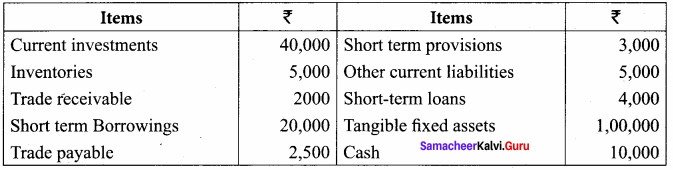Solution: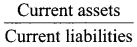Current assets = Current investments + Inventories + Trade receivables + Cash and cash + short – term loans and Advances
= ₹ 40,000 + 5,000 + 2,000 + 10,000 + 4,000 = ₹ 61,000
Current liabilities = Short – term borrowings + Trade payable + short-term provisions + other current liabilities
= ₹ 20,000 + 2,500 + 3,000 + 5,000 = ₹ 30,500
Current Ratio = $$\frac{61,000}{30,500}$$ = 2 : 1

(ii) Quick ratio
Quick ratio =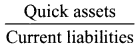Quick assets = Current assets – Inventories – Prepaid expenses
= ₹ 61,000 – 5,000 – Nil = ₹ 56,000
Quick Ratio = $$\frac{56,000}{30,500}$$ = 1.8 : 1

Question 2.
Current ratio = 2:1. State and give reason which of the following would improve, reduce or does not change the ratio
(a) Repayment of current liabilities
(c) Sale of office equipments for ₹ 4000 (book value ₹ 5000)
(d) Sale of goods ₹ 11,000 (cost ₹ 10,000)
(e) Payment of dividend
Current ratio of a company is 2:1. In other words, it may be assumed that the current assets are ₹ 2,00,000 and current liabilities are ₹ 1,00,000.
(a) Repayment of current liabilities say ₹ 50,000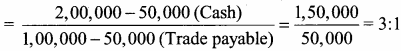Ratio is improved

(b) Purchasing goods on credit say ₹ 60,000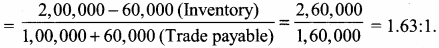Ratio reduced.

(c) Sale of office equipment for ₹ 4,000 (book value ₹ 5,000)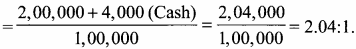Ratio is improved.

(d) Sale of goods ₹ 11,000 [Cost value is ₹ 10,000]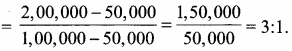Ratio is improved.

(e) Payment of dividend say ₹ 50,000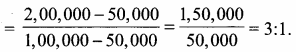= 3 : 1 Ratio is Improved

Question 3.
Calculate current ratio of a company from the following information
Inventory turnover ratio = 4 times
Inventory in the end was ₹ 20,000 more than inventory in the beginning. Revenue from operations ₹ 3,00,000 Gross profit ratio = 25%
Current liabilities ₹ 40,000; Quick ratio 0.75:1
Cost of revenue from operations
Inventory turnover ratio =4(Given) =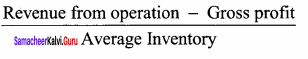Average Inventory =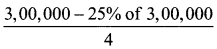Average inventory = $$\frac{2,25,000}{4}$$ = ₹ 56,250
Closing inventory = 56,250 + $$\frac{1}{2}$$ (20,000)
₹ 56,250 + 10,000 = ₹ 66,250
Current assets = Liquid assets + Closing inventory with the help of quick ratio. We can findout liquid assets.
Quick ratio =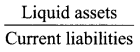0.75(given) =$$\frac{\text { Liquid assets }}{40,000}$$
Liquid assets = ₹ 40,000 x 0.75 = ₹ 30,000
Current assets = Liquid assets + Inventory
= ₹ 30,000 + ₹ 66,250 = ₹ 96,250
Current ratio == $$\frac{96,250}{40,000}$$ = 2.41:1

Question 4.
From the given balance sheet of Mr. A, calculate the activity (turnover) ratios.
Balance sheet as on 31.12.2004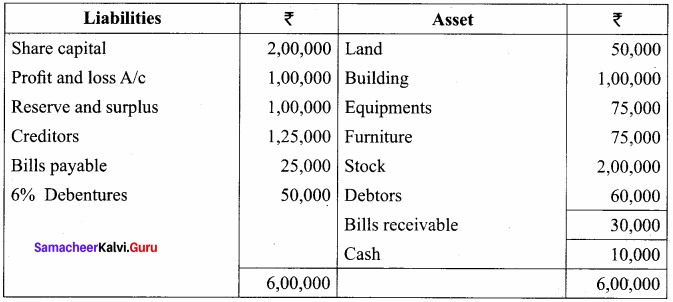Additional Information: Credit purchase ₹ 5,00,000; credit sales ₹ 9,00,000
(a) Capital Turnover Ratio =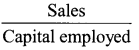Capital employed = Share capital + Profit and loss A/c + Reserve and surplus + debentures
= ₹ 2,00,000 + 1,00,000 + 1,00,000 + ₹ 50,000 = ₹ 4,50,000
Capital turnover ratio = $$\frac{9,00,000}{4,50,000}$$ = 2 times

(b) Fixed assets turnover ratio =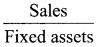Fixed assets = Land + Buildings + Equipments + Furniture = ₹ 50,000 + 1,00,000 + 1,75,000 + 75,000
= ₹ 3,00,000
Fixed assets turnover ratio = $$\frac{9,00,000}{4,50,000}$$

(c) Debtors Turnover ratio =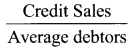Credit ₹ 9,00,000.
Average debtors = Closing debtors = ₹ 60,000
Debtors Turnover Ratio = $$\frac{9,00,000}{60,000}$$ = 15 times

(d) Creditors turnover ratio =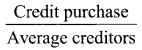Credit purchases = ₹ 5,00,000
Average creditors = closing creditors ₹ 1,25,000
Creditors turnover ratio = $$\frac{5,00,000}{1,25,00}$$ = 4 times

Question 5.
From the following particulars ascertain gross profit ratio:
Cash sales – ₹ 30,000
Gross profit – ₹ 1,58,500
Credit sales – ₹ 1,70,000
Return inward – ₹ 5,000
Gross profit = $$\frac{\text { Gross profit }}{\text { Sales }}$$ x 100
Sales = Cash sales + Credit sales – Sales return
= ₹ 30,000 + 1,70,000 – 5,000
= ₹ 1,95,000
Gross profit ratio = $$\frac{58,500}{1,95,000}$$ x 100 = 30%

Question 6.
Calculate current ratio
Cash ₹ 2,00,000; Sundry debtors ₹ 80,000
Stock ₹ 1,20,000; Sundry Creditors ₹ 1,50,000
Bills payable ₹ 50,000
Current ratio =Current Assets = Cash + Sundry debitor + Stock
= ₹ 2,00,000 + 80,000 + 1,20,000
= ₹ 4,00,000
= ₹ 1,50,000 + 50,000 = ₹ 2,00,000
Current ratio = $$\frac{4,00,000}{2,00,000}$$ = 2:1

Question 7.
Calculate Liquidity ratio
Current assets – ₹ 20,000
Prepaid expenses – ₹ 1,000
Stock – ₹ 3,000
Current liabilities – ₹ 8,000
Liquidity ratio =Liquid assets = Current assets – (stock + Prepaid exp)
= ₹ 20,000 – (3000+ 1000)
= ₹ 20,000 – 4000 = ₹ 16,000 Current liabilities = ₹ 8,000
Liquid Ratio = $$\frac{16,000}{8,000}$$ = 2:1

Question 8.
Calculate Debt – Equity ratio.
Equity share capital ₹ 2,00,000; General reserve ₹ 1,50,000; Long term loan Debt – Equity ₹ 50,000; Debenture ₹ 1,00,000.
Debt – Equity ratio =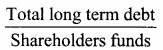Total long term debt = debentures + long term loan = ₹ 1,00,000 + ₹ 50,000 = ₹ 1,50,000
Shareholders fund = Equity share capital + General reserve
= ₹ 2,00,000 + ₹ 1,50,000
= ₹ 3,50,000
Debt – equity ratio = $$\frac{1,50,000}{3,50,000}$$ = 0.43 : 1

Question 9.
From the following calculate gross profit ratio:
Gross profit ₹ 50,000 Sales ₹ 5,50,000
Sales return = ₹ 50,000
= $$\frac{\text { Gross profit }}{\text { Sales }}$$ x 100
Sales = Sales – sales returns
= ₹ 5,50,000 – ₹ 50,000
= ₹ 5,00,000
Gross profit ratio = $$\frac{50,000}{5,00,000}$$ = 10%

Question 10.
Calculate gross profit ratio:
Sales ₹ 6,50,000; Cost of goods sold ₹ 4,80,000; Sales returns ₹ 50,000.
Gross profit ratio = $$\frac{\text { Gross profit }}{\text { Sales }}$$ x 100
Sales = Sales – Sales returns
= ₹ 6,50,000 – ₹ 50,000
= ₹ 6,00,000
Gross profit = Sales – Cost of goods sold
= ₹ 6,00,000 – 4,80,000
= ₹ 1,20,000
Gross profit ratio = $$\frac { 1,20,000 }{ 6,00,000 }$$ x 100 = 20%

Question 11.
Calculate capital turnover ratio:
Sales ₹ 20,40,000; Equity share capital ₹ 2,00,000
Preference share capital ₹ 1,00,000; Sales return ₹ 40,000
Loan ₹ 50,000; Reserve ₹ 50,000.
Capital turnover ratio =Sales = Sales – Sales returns
= ₹ 20,40,000 – ₹ 40,000
= ₹ 20,00,000
Capital employed = Equity share capitals + Preference share capital + Reserve + Loans
= ₹ 2,00,000 + 1,00,000 + 50,000 + 50,000
= ₹ 4,00,000
Capital turnover ratio = $$\frac { 20,00,000 }{ 4,00,000 }$$ = 5 times

Question 12.
From the following data, calculate the fixed assets turnover ratio: ₹ 6,00,000; fixed assets depreciation ₹ 2,00,000 Total sales ₹ 17,00,000; Sales returns ₹ 1,00,000.
Fixed assets turnover ratio =Sales = Total sales – Sales returns
= ₹ 17,00,000 – 1,00,000
= ₹ 16,00,0000
Fixed assets = Fixed assets – depreciation
= ₹ 6,00,000 – ₹ 2,00,000
= ₹ 4,00,000
Fixed assets turnover ratio = $$\frac { 16,00,000 }{ 4,00,000 }$$ = 4 times

Question 13.
Calculate Stock – Turnover ratio:
Opening stock = ₹ 80,000; Closing stock ₹ 60,000; Purchases ₹ 1,90,000.
Inventory turnover ratio =Average Inventory =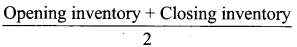= $$\frac{80,000+60,000}{2}$$ = $$\frac{1,40,000}{2}$$ = ₹ 70,000
Inventory Turnover Ratio = $$\frac{ 2,10,000 }{ 70,000 }$$ = 3 times